# 视频小教程_R语言中的批量操作

（本次操作有配套的视频教程，在果子学生信公众号回复“果子爱批量”自行获取，和代码一起以project的形式分享）

8秒完成2万个基因的生存分析，人人都可以！

### 1.lapply

``````lapply(as.list(1:4), log2)
``````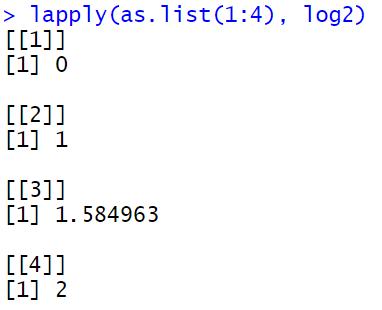``````lapply(1:4, log2)
``````

lapply的厉害之处在于，他作用的function可以自己写，那么这样一来就千变万化了。

``````lapply(1:4, function(x){
x^2 +2
})
``````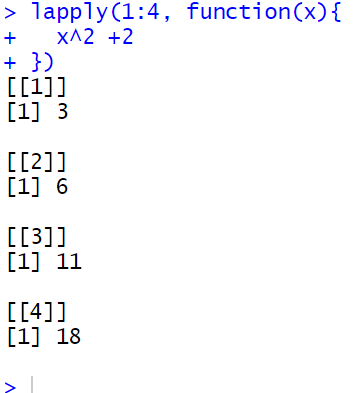### 2.do.call

do.call中的函数，需要能够接受多个参数。

cbind(), rbind(),c()都有这个特点。

``````sum(..., na.rm = FALSE)
c(...)
cbind(..., deparse.level = 1)
rbind(..., deparse.level = 1)
``````

lapply是list在前，函数在后

``````lapply(1:4, log2)
``````

do.call是函数在前，列表在后

``````dd <- lapply(1:4, log2)
``````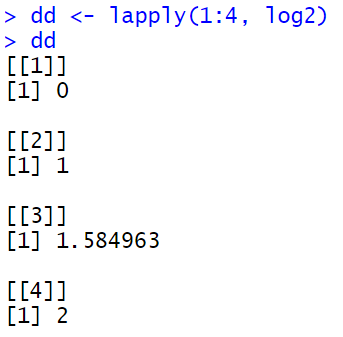``````do.call(c,dd)
``````

`````` 0.000000 1.000000 1.584963 2.000000
``````

### 3.lapply 和do.call 连用

``````dd <- lapply(LETTERS[1:6], function(i){
paste0(i,1:10)
})
``````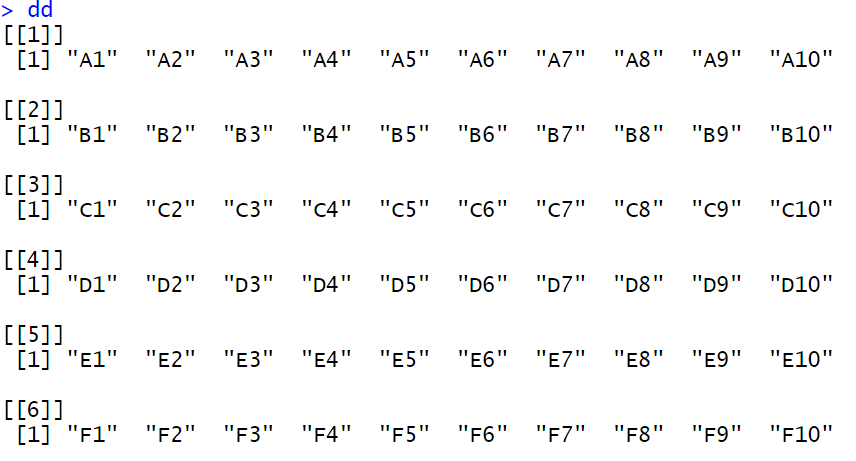``````do.call(rbind,dd)
``````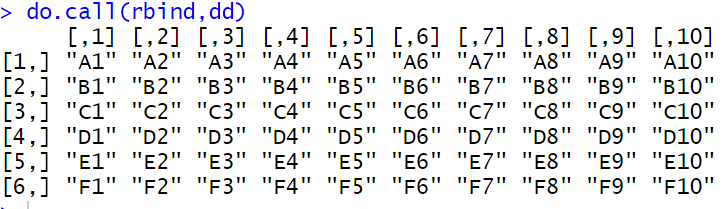``````do.call(cbind,dd)
``````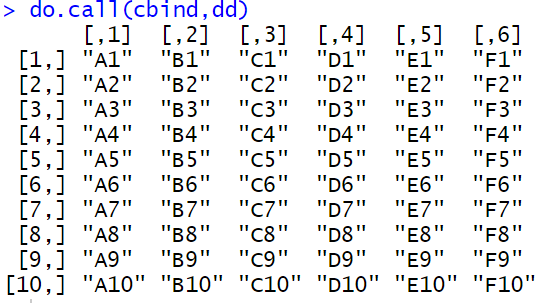R语言中性价比最高的函数以及最贵的函数

``````union(x, y)
intersect(x, y)
setdiff(x, y)
setequal(x, y)
``````

### 4.Reduce

`Reduce`函数就是一个解决方案。

Reduce函数实现多个数据框批量合并

lapply并行化处理

R语言中的批量分组操作